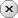### 用户注册### 用户登录### 发表随想# 实时折线图

2016-12-10 作者：13885620776举报

## [vb]代码库

```窗体代码,保存在 frm 文件里的.

'窗体代码,窗体上,只有一个按钮 command2 ,一个定时器 timer1 , 一个Picture1 , 一个标签 Label2 ,
'其中标签 是在 Picture1 中的. 标签的设置为 自动大小=true
Option Explicit
Dim 当前数据 As Double

Dim 目标数据 As Double

Dim 工作 As Boolean

Private Sub Command2_Click()
工作 = Not 工作
End Sub

Private Sub Form_Load()
工作 = False
End Sub

Private Sub Picture1_MouseMove(Button As Integer, Shift As Integer, X As Single, Y As Single)
'显示提示
Dim i As Long

'只判断左右移动,不判断上下
If 宽间格 > 0 Then
i = (X - 左边距) / 宽间格
If i > 0 And i < 数据个数Y + 1 Then

'这里是提示的内容,根据结构来写
'这里的,提示格式是: 编号,时间 ,下一行,值
Label2.Caption = i + 计数 - 1 & vbCrLf & 数据(i).时间 & vbCrLf & 数据(i).值
Label2.Move X - Label2.Height, Y - Label2.Width
Label2.Visible = True
Else
Label2.Visible = False
End If
Else
Label2.Visible = False
End If
End Sub

Private Sub Timer1_Timer()

'根据数据的产生

Dim 速度 As Double

If 工作 Then

速度 = Rnd()

If Abs(当前数据 - 目标数据) < 0.5 Then
目标数据 = Round(Rnd() * 11 + 3, 2)
End If

If 当前数据 > 目标数据 Then
当前数据 = 当前数据 - 速度
Else
当前数据 = 当前数据 + 速度
End If
当前数据 = Round(当前数据, 2)

If 当前数据 > 14 Then
当前数据 = 14
End If
If 当前数据 < 3.5 Then
当前数据 = 3.5
End If

Call ADD数据(Time, 当前数据)
Call 绘折线图(Picture1)

End If

End Sub

'模块代码
Option Explicit

'这个结构里需要使用到的数据 为 值 , 而 X,Y 是计算出来的, 其它可以用于提示里面,也可以不要.
Public Type 数据结构类型
时间 As Date
值  As Double
X As Long       '屏幕提示用的
Y As Long
End Type

'折线图 坐标个数
Public Const 数据个数Y = 30
Public Const 数据个数X = 12          '等你期望的分格数+2, ,如分为10格,那么这里就填12,下面要各空一格

Public 数据(1 To 数据个数Y) As 数据结构类型
Public Max值 As Double
Public Min值 As Double
Public 高间格 As Long, 宽间格 As Long

Public Const 坐标颜色 = 0
Public Const 网格颜色 = vbGreen
Public Const 折线颜色 = vbRed
Public Const 标注颜色 = 3
Public Const 左边距 = 400

Public 计数 As Long

Public Sub ADD数据(cs1 As String, cs2 As Double)
Dim i As Long
If IsDate(cs1) And IsNumeric(cs2) Then
For i = 2 To 数据个数Y
数据(i - 1).时间 = 数据(i).时间
数据(i - 1).值 = 数据(i).值
'数据(i - 1).X = 数据(i).X
'数据(i - 1).Y = 数据(i).Y
Next i
数据(数据个数Y).时间 = cs1
数据(数据个数Y).值 = cs2

'如果Y坐标值不需要变的话,那么下面这行就不要.
计数 = 计数 + 1
End If
'X Y需要重新计算,所以不需要移动
End Sub

Public Sub MaxMin值()       '找出最大值,最小值

'根据当前数据动态调整坐标
'Dim i As Long
'Max值 = 数据(1).值
'Min值 = 数据(1).值
'For i = 2 To 50
'    If Max值 < 数据(i).值 Then
'        Max值 = 数据(i).值
'    End If
'    'If Min值 > 数据(i).值 Then
'    '    Min值 = 数据(i).值
'    'End If
'Next i

'这里是坐标大小
Max值 = 15
Min值 = 3

End Sub

Public Sub Cls数据()

Dim i As Long
For i = 1 To 数据个数Y
数据(i).值 = 0
数据(i).时间 = #12:00:00 AM#
Next i

End Sub

Public Sub 读数据(cs As String)
'例,按这个结构来的

Dim fr As Long
fr = FreeFile

Dim d As String
Dim fj() As String
Dim j As String

Open cs For Input Access Read As #fr
Do While Not EOF(fr)
Line Input #fr, j
If InStr(1, j, ";") > 0 Then
fj = Split(j, ";")
If d <> fj(0) Then
d = fj(0)
Call ADD数据(fj(0), CDbl(fj(1)))
End If
End If
Loop

Close fr
End Sub

Public Sub 绘折线图(cs As PictureBox)

Dim i As Long, 间格 As Double
Dim 总高 As Long
Dim 最低格 As Double

With cs

Call MaxMin值           '找出最大值,最小值
If Min值 = 0 Then
间格 = (Max值) / (数据个数X + 1)    '分格,上下各空一格,为0时,下面不用空
最低格 = 0
Else
间格 = (Max值 - Min值) / 数据个数X     '分格,
最低格 = Min值
End If

.Cls      '清屏

'画坐标
Dim x1 As Long, X2 As Long, y1 As Long, y2 As Long

y1 = .ScaleHeight - 200
x1 = .ScaleWidth - 200

cs.Line (左边距, 0)-(左边距, y1), 坐标颜色
cs.Line (左边距, y1)-(.ScaleWidth, y1), 坐标颜色

'画坐标网络
.ForeColor = 标注颜色
.CurrentX = 0
.CurrentY = y1 - 90
cs.Print Round(最低格, 3)
For i = 1 To 数据个数X - 1
cs.Line (左边距, y1 - i * 高间格)-(.ScaleWidth, y1 - i * 高间格), 网格颜色
.CurrentX = 0
.CurrentY = y1 - i * 高间格 - 90
cs.Print Round(Min值 + i * 间格, 3)
Next i

For i = 1 To 数据个数Y
cs.Line (左边距 + i * 宽间格, 0)-(左边距 + i * 宽间格, y1), 网格颜色
.CurrentX = 左边距 + i * 宽间格 - 150
.CurrentY = y1 + 30
cs.Print i + 计数 - 1
Next i

'画折线图
数据(1).Y = y1 - ((数据(1).值 - 最低格) / (Max值 - 最低格)) * 总高
数据(1).X = 左边距 + 宽间格
cs.Circle (数据(1).X, 数据(1).Y), 30, 折线颜色
For i = 2 To 数据个数Y
数据(i).Y = y1 - ((数据(i).值 - 最低格) / (Max值 - 最低格)) * 总高
数据(i).X = 左边距 + (i) * 宽间格
cs.Circle (数据(i).X, 数据(i).Y), 30, 折线颜色
cs.Line (数据(i - 1).X, 数据(i - 1).Y)-(数据(i).X, 数据(i).Y)
Next i

End With
End Sub```

### 网友评论    (发表评论)

• by:        发表于：2017-12-01 14:55:59

??

### 回复评论还能输入：1000字

### 评论须知：

• 1、评论每次加2分，每天上限为30；
• 2、请文明用语，共同创建干净的技术交流环境；
• 3、若被发现提交非法信息，评论将会被删除，并且给予扣分处理，严重者给予封号处理；
• 4、请勿发布广告信息或其他无关评论，否则将会删除评论并扣分，严重者给予封号处理。